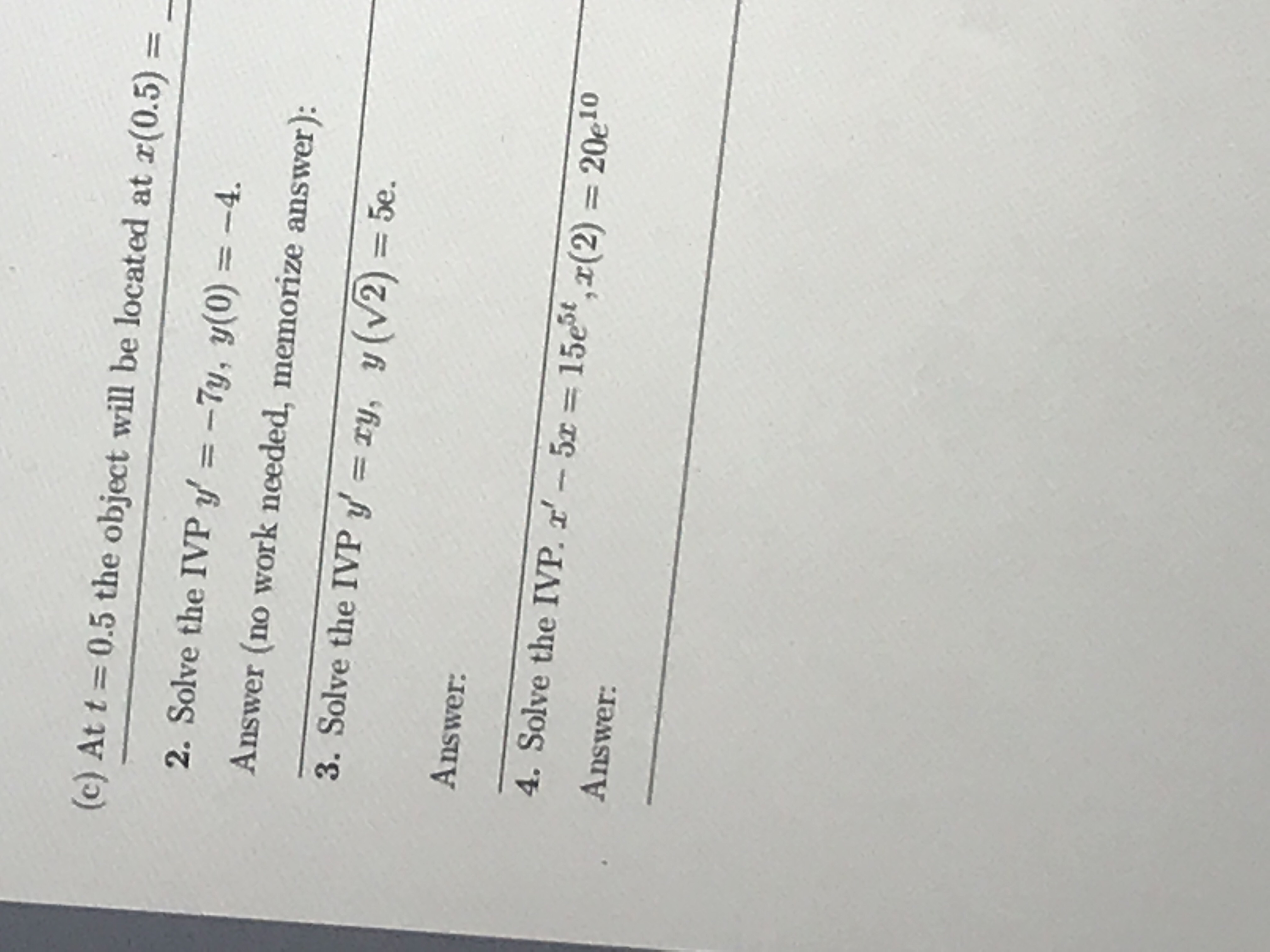# (c) At t 0.5 the object will be located at r(0.5)- 2. Solve the IVPy'=-7y, y(0)=-4. Answer (no work nceded, memorize answer): 3. Solve the IVPy-xy, y (V2) = 5e. Answer: 4. Solve the IVP, 2'-5x = 15e5ta(2) Answer: 20eio

Question

3help_outlineImage Transcriptionclose(c) At t 0.5 the object will be located at r(0.5)- 2. Solve the IVPy'=-7y, y(0)=-4. Answer (no work nceded, memorize answer): 3. Solve the IVPy-xy, y (V2) = 5e. Answer: 4. Solve the IVP, 2'-5x = 15e5ta(2) Answer: 20eio fullscreen